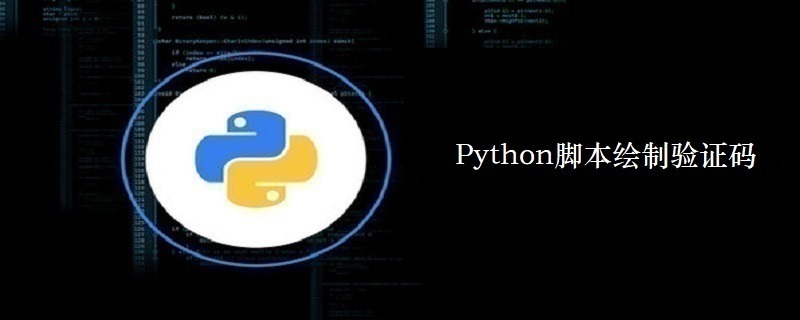## Python脚本绘制验证码

224次阅读

PIL：Python Imaging Library，已经是Python平台事实上的图像处理标准库了。PIL功能非常强大，但API却非常简单易用。```# -*- coding: utf-8 -*-
#导入三个模块
import Image,ImageDraw,ImageFont
import random
import math
'''基本功能'''
#图片宽度
width = 100
#图片高度
height = 40
#背景颜色
bgcolor = (255,255,255)
#生成背景图片
image = Image.new('RGB',(width,height),bgcolor)
#加载字体
font = ImageFont.truetype('FreeSans.ttf',30)
#字体颜色
fontcolor = (0,0,0)
#产生draw对象，draw是一些算法的集合
draw = ImageDraw.Draw(image)
#画字体,(0,0)是起始位置
draw.text((0,0),'1234',font=font,fill=fontcolor)
#释放draw
del draw
#保存原始版本
image.save('1234_1.jpeg')
'''演示扭曲，需要新建一个图片对象'''
#新图片
newImage = Image.new('RGB',(width,height),bgcolor)
offset = 0
for y in range(0,height):
offset += 1
for x in range(0,width):
#新的x坐标点
newx = x + offset
#你可以试试如下的效果
#newx = x + math.sin(float(y)/10)*10
if newx < width:
#把源像素通过偏移到新的像素点
newPix[newx,y] = pix[x,y]
#保存扭曲后的版本
newImage.save('1234_2.jpeg')
'''形变一下'''
#x1 = ax+by+c
#y1 = dx+ey+f
newImage = image.transform((width+30,height+10), Image.AFFINE, (1,-0.3,0,-0.1,1,0))
newImage.save('1234_3.jpeg')
'''画干扰线，别画太多，免得用户都看不清楚'''
#创建draw，画线用
draw = ImageDraw.Draw(newImage)
#线的颜色
linecolor= (0,0,0)
for i in range(0,15):
#都是随机的
x1 = random.randint(0,width)
x2 = random.randint(0,width)
y1 = random.randint(0,height)
y2 = random.randint(0,height)
draw.line([(x1, y1), (x2, y2)], linecolor)
#保存到本地
newImage.save('1234_4.jpeg')```